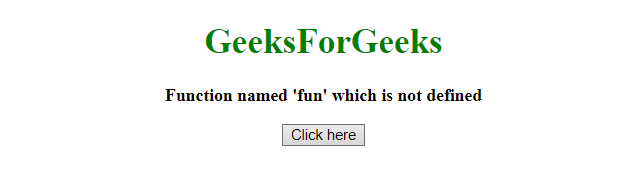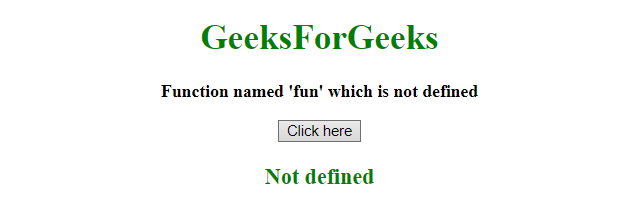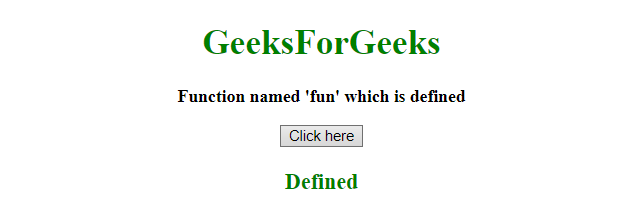# How to check a function is defined in JavaScript ?

• Last Updated : 24 May, 2019

The job is to identify whether a function is defined or not. The JavaScript typeof operator is used to solve the problem described below:

Javascript typeof Operator: This operator can be used to find the type of a JavaScript variable. This operator returns the type of a variable or an expression:

Hey geek! The constant emerging technologies in the world of web development always keeps the excitement for this subject through the roof. But before you tackle the big projects, we suggest you start by learning the basics. Kickstart your web development journey by learning JS concepts with our JavaScript Course. Now at it's lowest price ever!

Syntax:

`typeof var`

Parameter: It contains single value var which is a Javascript variable.

Return value: It returns the type of a variable or an expression:

Example 1: This example checks the type of the function, If it is function then it is defined otherwise not defined by using typeof operator.

 ` ``<``html``> ``    ``<``head``> ``        ``<``title``> ``            ``How to check a function is defined``        ````    `` ``     ` `    ``<``body` `style` `= ``"text-align:center;"``> ``     ` `        ``<``h1` `style` `= ``"color:green;"` `> ``            ``GeeksForGeeks ``        `` ``         ` `        ``<``p` `id` `= ``"GFG_UP"` `style` `= ``"font-size: 16px; font-weight: bold;"``>``        ````         ` `        ``<``button` `onclick` `= ``"gfg_Run()"``> ``            ``Click here``        ````         ` `        ``<``p` `id` `= ``"GFG_DOWN"` `style` `= ``            ``"color:green; font-size: 20px; font-weight: bold;"``>``        ````         ` `        ``<``script``>``            ``var el_up = document.getElementById("GFG_UP");``            ``var el_down = document.getElementById("GFG_DOWN");``             ` `            ``el_up.innerHTML = ``                ``"Function named 'fun' which is not defined";``                 ` `            ``function gfg_Run() {``                ``var defined = 'Not defined';``                ``if(typeof fun == 'function') {``                    ``defined = "Defined";``                ``}``                ``el_down.innerHTML = defined;``            ``}         ``        `` ``    `` ``                    `

Output:

• Before clicking on the button:• After clicking on the button:Example 2: This example checks the type of the function, If it is function then it is defined otherwise not defined by using typeof operator by creating a function.

 ` ``<``html``> ``    ``<``head``> ``        ``<``title``> ``            ``How to check a function is defined``        ````    `` ``     ` `    ``<``body` `style` `= ``"text-align:center;"``> ``     ` `        ``<``h1` `style` `= ``"color:green;"` `> ``            ``GeeksForGeeks ``        `` ``         ` `        ``<``p` `id` `= ``"GFG_UP"` `style` `= ``"font-size: 16px; font-weight: bold;"``>``        ````         ` `        ``<``button` `onclick` `= ``"gfg_Run()"``> ``            ``Click here``        ````         ` `        ``<``p` `id` `= ``"GFG_DOWN"` `style` `= ``            ``"color:green; font-size: 20px; font-weight: bold;"``>``        ````         ` `        ``<``script``>``            ``var el_up = document.getElementById("GFG_UP");``            ``var el_down = document.getElementById("GFG_DOWN");``             ` `            ``el_up.innerHTML = "Function named 'fun' which is defined";``             ` `            ``function isFunction(possibleFunction) {``                ``return typeof(possibleFunction) === typeof(Function);``            ``}``             ` `            ``function fun() {``                 ` `            ``}``             ` `            ``function gfg_Run() {``                ``var defined = 'Not defined';``                ``if(isFunction(fun)) {``                    ``defined = "Defined";``                ``}``                ``el_down.innerHTML = defined;``            ``}         ``        `` ``    `` ``                    `

Output:

• Before clicking on the button:• After clicking on the button:<

My Personal Notes arrow_drop_up